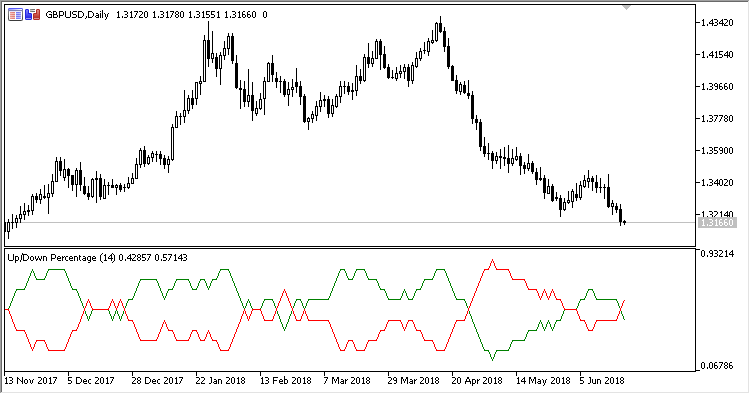# UDP – indicator for MetaTrader 5

• A+
category：MT5 INDICATORS

Indicator UDP (Up/Down Percentage) displays the direction of candlesticks, i.e., up/down, as a percentage over the selected period.

It has two configurable parameters:

• Period - calculation period;
• Applied price - price used for calculations.

Calculations:

```UP = SMA(UpF, Period)
DN = SMA(DnF, Period)
```

where:

If Price > PrevPrice:

`UpF = 1`

otherwise:

`UpF = 0`

If Price < PrevPrice:

`DnF = 1`

otherwise:

`DnF = 0`